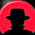Saturday, January 12, 2013

Inverse Kinematics for 3 DOF Hexapod Made Easy

As defined by Wikipedia, inverse kinematics is 'the use of the kinematics equations of a robot to determine the joint parameters that provide a desired position of the end-effector.' In other words, the purpose of inverse kinematics (IK) is to take X,Y,Z coordinates and produce angles for all your motors with the use of trigonometric formulas. The formulas I'll be working out will be used for 3 DOF hexapod robots like the Lynxmotion Phoenix as seen below; though, my formulas and methods can be adapted to work for other designs of hexapods, octopods, quadropods, or anything with legs really.

The first step is to start assigning values and variables for different angles and lengths on the legs. I've drawn (vary) rough diagrams for reference and better understanding when working the formulas.

Now, intuition would tell you to first solve the forward kinematics then work the formula backward. This will not work though. The variables can not be isolated by reversing the forward kinematics. Fortunately for us, the nature of the hexapods legs makes solving the kinematics surprisingly simple. Although, in all honesty, it was a puzzle that took me weeks to solve so don't feel too bad if you've had trouble with the problem yourself. So now it's time to get creative. I won't walk you through the full process i went through to find the solution to this problem (partially because i don't exactly know) so we will just jump into the actual solution.

first we find the overall length of the leg segments in two dimensions in reference to the top view (which we will assign the value G). if that's confusing then refer to this diagram:
clearly to find G we need to use the Pythagorean theorem. so: G=√X²+Z²

Now we'll switch to the side view and solve for H:
Again, we will use the Pythagorean theorem. so: H=√Y²+G²

Now we're going to focus on triangle ABH.All side lengths of triangle ABH are now known so we can use our humble friend, the Law Of Cosines to find angle b and then use the Law Of Sines to find angle h.
the law of cosines: [c² = a² + b² - 2ab cosC] or in our triangle [B²=A²+H²-2AH(cos b)]

if we rework that to solve for angle b we get:  b = acos((A²+H²-B²)/2AH)

Now we solve for h with the Law of Sines. the law of sines states: sin(h)/H = sin(b)/B
again, we solve for h and we get: h=asin(H(sin(b))/B)

We now have all the angles we need in this triangle but they are not as we want them h is 180° higher then angle 2 so we have to adjust: angle 2= h-180

Angle 1 is a little more complicated. We need to add angle b and angle g then subtract 90°
Of course to do this we need to first find g which is simply: g=atan(G/Y)
so: angle 1=(atan(G/Y))+b-90

Great, now we have angle 1 and angle 2 but OH NO. we've left out angle 3. don't worry, this is the easiest of all of the calculations. we have several options since X(tan(angle 3))=Z, G(cos(angle 3))=X, and G(sin(angle 3))=Z. Feel free to pick whichever you want and solve for angle 3 but i will pick the first. We solve for angle 3 and we get: angle 3=atan(X/Z)

Formulas Used In Order
G=√X²+Z²
H=√Y²+G²
b = acos((A²+H²-B²)/2AH)
h=asin(H(sin(b))/B)
angle 2= h-180
angle 1=(atan(G/Y))+b-90
angle 3=atan(X/Z)

Condensed Formulas for Motor Angles 1,2,3

angle 1=(atan((√X²+Z²)/Y))+[acos((A²+[√Y²+(√X²+Z²)]²-B²)/2A[√Y²+(√X²+Z²)])]-90

angle 2= [asin([√Y²+(√X²+Z²)](sin([acos((A²+[√Y²+(√X²+Z²)]²-B²)/2A[√Y²+(√X²+Z²)])]))/B)]-180

angle 3=atan(X/Z)

1.Thanks verry much, it was verry helpfull !!!

2.cannot calculate the angle 2, does any able to get the angle 2?

3.4.5.6.